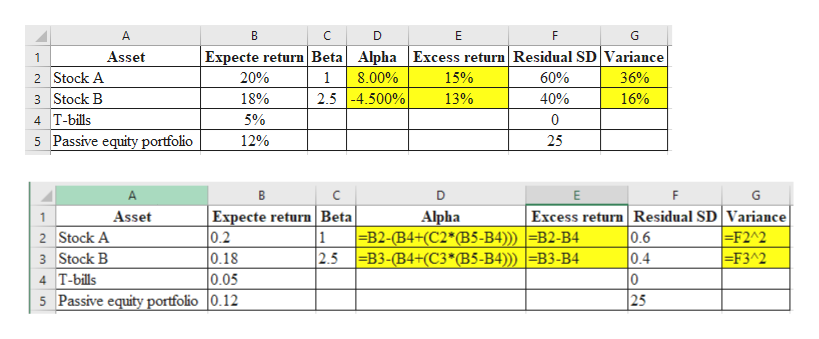# A portfolio manager summarizes the input from the macro and micro forecasts in the following table:Micro ForecastsAssetExpected Return (%)BetaResidual Standard Deviation (%)Stock A1.002060Stock B2.501840Macro ForecastsExpected Return (%)Standard Deviation (%)AssetT-bills5оPassive Equity Portfolio (m)1225a. Calculate expected excess returns, alpha values, and residual variances for these stocks.Instruction: Enter your answer as a percentage (rounded to two decimal places) for expected excess returns and alpha values.Expected excess return on stock AExpected excess return on stock BAlpha of stock AAlpha of stock BInstruction: Enter your answer as a decimal number rounded to two decimal places for residual variances.Residual variance of stock AResidual variance of stock BInstruction: for part b, enter your response as a decimal number rounded to four decimal places.b. Suppose that the portfolio manager follows the Treynor-Black model, and constructs an active portfolio (p) that consists of the abovetwo stocks. The alpha of the active portfolio (p) is -18%, and its residual standard deviation is 150%What is the Sharpe ratio for the optimal portfolio (consisting of the passive equity portfolio and the active portfolio (p)? Micro ForecastsResidual Standard Deviation (%AssetExpected Return (%)BetaStock A201.0060Stock B182.5040Macro ForecastsExpected Return (%)AssetStandard Deviation (%)O0T-billsPassive Equity Portfolio (m)1225a. Calculate expected excess returns, alpha values, and residual variances for these stocks.Instruction: Enter your answer as a percentage (rounded to two decimal places) for expected excess returns and alpha values.Expected excess return on stock AExpected excess return on stock BAlpha of stock AAlpha of stock BInstruction: Enter your answer as a decimal number rounded to two decimal places for residual variances.Residual variance of stock AResidual variance of stock BInstruction: for part b, enter your response as a decimal number rounded to four decimal places.b. Suppose that the portfolio manager follows the Treynor-Black model, and constructs an active portfolio (p) that consists of the abovetwo stocks. The alpha of the active portfolio (p) is -18%, and its residual standard deviation is 150%.What is the Sharpe ratio for the optimal portfolio (consisting of the passive equity portfolio and the active portfolio (p)?What's the M2 of the optimal portfolio?

Question
45 views

Need help on all

check_circle

Step 1

Hi there, Thanks for posting the question. As per Q&A Guidelines, we should answer the first question when multiple questions posted under single question. Hence, I have answered part A below. Please repost the other questions separately. Thank you.

Step 2

Calculate the excess returns, alpha, an...help_outlineImage TranscriptioncloseA В C E G Expecte return Beta Alpha Excess return Residual SD Variance Asset 1 2 Stock A 3 Stock B 4 T-bills 5 Passive equity portfolio 20% 1 8.00% 15% 60% 36% 2.54.500% 40% 18% 13% 16% 5% 0 25 12% В E Excess return Residual SD Variance 0.6 0.4 0 25 Expecte return Beta 0.2 0.18 0.05 Alpha Asset 1 1 |=B2-(B4+(C2*(B5-B4)) =B2-B4 =F2A2 =F3^2 2 Stock A 3 Stock B 2.5 B3-(B4+(C3*(B5-B4))) =B3-B4 4 T-bills 5 Passive equity portfolio 0.12 co fullscreen

### Want to see the full answer?

See Solution

#### Want to see this answer and more?

Solutions are written by subject experts who are available 24/7. Questions are typically answered within 1 hour.*

See Solution
*Response times may vary by subject and question.
Tagged in